# Chapter 12: Liquid-Liquid and Fluid Solid Separation Processes¶

## Example 12.1.1 Page Number 699¶

In :
#Adsorption  Isotherm for Phenol in Wastewater
import numpy as np
from scipy.optimize import curve_fit
import matplotlib.pyplot as plt

#Variable Declaration
c = np.array([0.322,0.117,0.039,0.0061,0.0011])         #kg Phenol per m3 solution
q = np.array([0.150,0.122,0.094,0.059,0.045])           #kg Phenol per kg Carbon

#Langmuir Isotherm Fitting function
def fit_func(x, q0, K):
return q0*c/(K+c)

params = curve_fit(fit_func, c, q)
[q0, K] = params

#Results
plt.grid(True, which='both')
print 'Langmuir Isotherm Fitting parameters are '
print "q0  = ", round(q0,3) ," K = ", round(K,3)

qi = q0*c/(K+c)
plt.figure(1)
plt.title('Langmuir Isotherm Fit giving a poor fit')
plt.xlabel('c, kg phenol/m3 waste water')
plt.plot(c,q,'ro-',label='Expt. Data')
plt.plot(c,qi,'bo-',label='Fitted Data')
plt.legend(loc = 'lower right')

#Freundlich Isotherm Fitting function
def fit_func(c, K, n):
return K*c**n

params = curve_fit(fit_func, c, q)
[K, n] = params

qi = K*c**n

plt.figure(2)
plt.grid(True, which='both')
plt.title('Freundlich Isotherm Fitting')
plt.xlabel('c, kg phenol/m3 waste water')

plt.loglog(c,q,'ro-',basex=10,basey=10,label='Expt. Data')
plt.loglog(c,qi,'bo-',basex=10,basey=10,label='Fitted Data')
plt.legend(loc = 'best')
plt.plot()

#plt.LogFormatterExponent(base=10)
#Results
print 'Freundlich Isotherm Fitting parameters are '
print "K  = ", round(K,3) ," n = ", round(n,3)

Langmuir Isotherm Fitting parameters are
q0  =  0.134  K =  0.007
Freundlich Isotherm Fitting parameters are
K  =  0.194  n =  0.223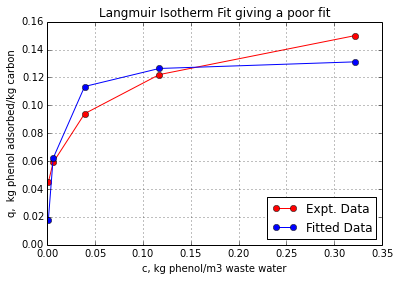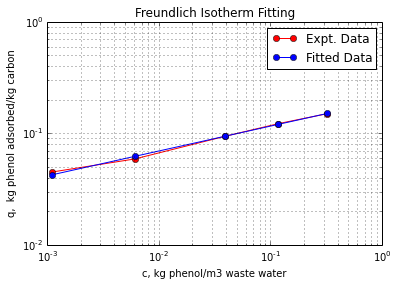## Example 12.2.1 Page Number 700¶

In :
#Adsorption  Isotherm for Phenol in Wastewater
import numpy as np
from scipy.optimize import root
import matplotlib.pyplot as plt

#Variable Declaration
M = 1.4                  #Mass of activated carbon in kg
S = 1.0                  #Surface area of carbon in m2
cF = 0.21                #Concentratio of phenol in feed in kg/m3
K = 0.194020642969       #Freundlich proportionality parameter
n = 0.222977691192       #Freundlich exponential parameter

#Calculations
#Amount adsorbed + Amount left in equilibrium solution = Amount fed with solution
#M*K*c**n + cF*S = c*S
c = arange(0,0.2,0.00001)
q = K*c**n
q1 = (cF-c)*S/M
plt.grid(True)
plt.plot(c,q1,'r-',c,q)
plt.xlabel('c, kg phenol/m3 waste water')
f = lambda x: M*K*x**n + x - cF*S
sol = root(f, 0.1)
ce = sol.x
qe = K*ce**n
plt.text(ce+0.01,qe,'Solution')
plt.text(ce+0.05,qe+0.02,'Isotherm')
plt.text(ce+0.05,qe-0.03,'Mass Balance')
plt.plot([0.0,ce,ce],[qe,qe,0.0])
plt.plot(ce, qe,'bo')
x =str(round(ce,4))
plt.text(ce,0.02,'c =' + x)
x =str(round(qe,4))
plt.text(0.001,qe + 0.005,'q =' + x)
ext = (cF-ce)*100/cF

#Results
print "Phenol equilibrium amount adsorbrd = ", round(qe,4) , "kg Phenol/kg carbon"
print "Phenol equilibrium amount in solution = ", round(ce,4) , "kg Phenol/m3 solution"
print 'Precent Phenol extracted = %3.1f'%(ext), "%"

Phenol equilibrium amount adsorbrd =  0.1048 kg Phenol/kg carbon
Phenol equilibrium amount in solution =  0.0632 kg Phenol/m3 solution
Precent Phenol extracted = 69.9 %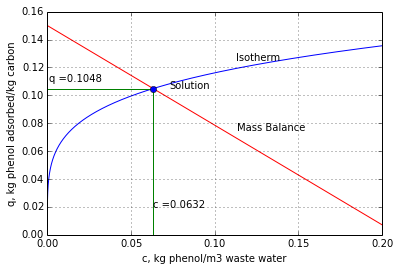## Example 12.3.1 Page Number 704¶

In :
#Scaleup of Laboratory Adsorption Column
import numpy as np
from scipy.optimize import curve_fit, root
import matplotlib.pyplot as plt
from scipy.interpolate import interp1d

#Variable Declaration
d = 4.                #Diameter of particle in cm
h = 14.               #Length of the bed in cm
m = 79.2              #Mass of carbon in gm
c0 = 600              #Inlet concentration of alcohol in ppm
rho = 0.00115         #Density of air in g/cc
Q = 754.              #Flowrate of air (cc/s)
cbyc0brk = 0.01       #Ratio of concentration at break point
tbb = 6.0             #Break point for new coloumn in hr

#Calculations
t = np.array([0.0,3.0,3.5,4.0,4.5,5.0,5.5,6.0,6.2,6.5,6.8])
cbyc0 = np.array([0.0,0.0,0.002,0.030,0.155,0.396,0.658,0.903,0.933,0.975,0.993])

def bisection(a,b,tol):
c = (a+b)/2.0
while (b-a)/2.0 > tol:
if f2(c) == 0:
return c
elif f2(a)*f2(c) < 0:
b = c
else :
a = c
c = (a+b)/2.0
return c

f = interp1d(t, cbyc0, kind='cubic',bounds_error=False)
plt.grid(True)
plt.plot(t,cbyc0,'ro')
tt = np.arange(0.0,7.,0.01)
qi =f(tt)
plt.plot(tt,qi,'b-')
plt.fill_between(t,cbyc0,1.,color='0.9')
plt.xlabel('$time, h$')
plt.ylabel('$c/c_0$')
plt.ylim(0.,1.)
plt.xlim(0.,7.)
#PART A
f2 = lambda t: f(t)-cbyc0brk
tb = bisection(0,4.,0.0001)

f3 = lambda t: 1-f(t)

LUB = h*tu/tt
LUNB =h*(1-tu/tt)
plt.plot([tb,tb],[0.0,1.],'--')
plt.plot([0.,tb],[0.01,.01],'--')

#Results PART A
print "Results for PART A"
print "Break point time for c/c0=0.01:",round(tb,2),"hr"
print "Time for usable capacity of bed:", round(tu,2), "hr"
print "Time for complete bed length utilization", round(tt,2),"hr"
print "Length of used bed:",round(LUB,2),"cm"
print "Length of unused bed:",round(LUNB,2),"cm"

#PART B
print
print "Results for PART B"
LUBB = tbb*LUB/tb
print "Length of used bed:",round(LUBB,2),"cm"
LBB = LUBB+LUNB
print "Length of unused bed:",round(LUNB,2),"cm"

mdot = Q*rho*3600.
fracBU = LUBB/LBB

print "Air flow rate:", round(mdot,2),"g air/hr"
print "Saturation Capacity:", round(Csat,3), "g alcohol/g carbon"
print "Fraction of new bed used:", round(fracBU,3)
print 'Length of bed %4.1f cm'%LBB

 Results for PART A
Break point time for c/c0=0.01: 3.78 hr
Time for usable capacity of bed: 3.84 hr
Time for complete bed length utilization 5.26 hr
Length of used bed: 10.23 cm
Length of unused bed: 3.77 cm

Results for PART B
Length of used bed: 16.25 cm
Length of unused bed: 3.77 cm
Air flow rate: 3121.56 g air/hr
Saturation Capacity: 0.124 g alcohol/g carbon
Fraction of new bed used: 0.812
Length of bed 20.0 cm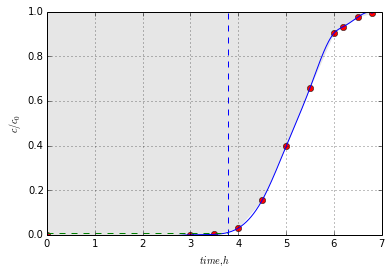## Example 12.5-1, Page Number 711¶

In :
#Material Balance for Equilibrium Layers

#Variable Declaration
mc = 30.              #Mass of isopropy ether in orignal mixture ,kg
ma = 10.              #Mass of acetic acid in orignal mixture ,kg
mb = 60.              #Mass of water in orignal mixture ,kg

#Calculations
m = ma+mb+mc
xma = ma/m
xmb = mb/m
xmc = mc/m

#Extract layer composition from Figure 12.5-3
ya = 0.04
yc = 0.94
yb = 1.- ya - yc
#Raffinate layer composition from Figure 12.5-3
xa = 0.12
xc = 0.02
xb = 1.0 - xa - xc

#Results
print "Composition of the orignal mixture for A,B,C reaspectively is ",xma,xmb,xmc
print "Raffinate layer compositions of A, B, C reaspectively are", xa,xb,xc
print "Extract layer compositions of A, B, C reaspectively are", ya,yb,yc

Composition of the orignal mixture for A,B,C reaspectively is  0.1 0.6 0.3
Raffinate layer compositions of A, B, C reaspectively are 0.12 0.86 0.02
Extract layer compositions of A, B, C reaspectively are 0.04 0.02 0.94


## Example 12.5-2, Page Number 714¶

In :
#Amounts of phases in Solvent Extraction

from numpy import linalg
#Variable Declaration
#From Example 12.5-
M = 100                  #Mass of activated carbon in kg
yA = 0.04
xA = 0.12
xAM = 0.1
#Calculation
#Balance on A in Feed
#    yA*V + xAL = 0.01M
#       V +   L =     M
a = np.array([[yA,xA], [1,1]])
b = np.array([xAM*M,M])
[V, L] = np.linalg.solve(a, b)

#Results
print 'Kg of extract phase: %3.1f \nKg of Raffinate phase: %3.1f'%(V,L)

#from figure 12.5-3
hg = 4.2
gi = 5.8
L = hg*M/gi
V = M-L

#Results from Graph

print 'from Graph'
print 'Kg of extract phase: %3.1f \nKg of Raffinate phase: %3.1f'%(V,L)

Kg of extract phase: 25.0
Kg of Raffinate phase: 75.0
from Graph
Kg of extract phase: 27.6
Kg of Raffinate phase: 72.4


## Example 12.7-1 Page Number 718¶

In :
#Material Balance for Counterurrent Stage Process
from numpy import linalg

#Variable Declaration
Vn1 = 600        #Ispropyl ether (C pure) rate, kg/hr
Lo = 200         #Feed rate, kg/hr
xAL = 0.30       #Wt fraction of Acetic acid A in Feed
yCn1 = 1.0       #Wt fraction of Ether C in solvent feed
xCo = 0.0        #Wt fraction of Ether C in Feed
yAn1 = 0.0       #Wt fraction of Acetic acid solvent feed
#Calculation
M = Vn1 + Lo
xCM = (Vn1*yCn1 + Lo*xCo)/M
xAM = (Vn1*yAn1 + Lo*xAL)/M

yA1 = 0.08
yC1 = 0.9
xCn = 0.017
a = np.array([[1,1], [0.017,0.9]])
b = np.array([M,M*xCM])
[Ln,V1] = np.linalg.solve(a, b)
#Results
print "Co-ordinates for the mixed feed are", xCM, xAM
print "Line passing through this intersect phase boundary at yA1 = 0.08 and yC1 = 0.9"
print "and xCn = 0.017 this is obtained from fig 12.7-3"
print "The amount of Raffinate", round(Ln,0), "kg/hr"
print "The amount of Extract", round(V1,0), "kg/hr"

Co-ordinates for the mixed feed are 0.75 0.075
Line passing through this intersect phase boundary at yA1 = 0.08 and yC1 = 0.9
and xCn = 0.017 this is obtained from fig 12.7-3
The amount of Raffinate 136.0 kg/hr
The amount of Extract 664.0 kg/hr


## Example 12.7-3 Page Number 722¶

In :
#Extraction of Nicotine with Immiscible Liquids
import numpy as np
from scipy.interpolate import interp1d
from scipy.optimize import root
import matplotlib.pyplot as plt

#Variable Declaration
x = np.array([0,0.001010, 0.00246,0.005,0.00746,0.00988,0.0202])        #Equilibrium Data
y = np.array([0,0.000806, 0.001959,0.00454,0.00682,0.00904,0.0185])
Lo = 100     #Feed rate, kg/hr
xo = 0.01    #Nicotine concentration in liquid feed, wt fraction
yn1 = 0.0005 #Nicotine concentration in solvent, wt fraction
xn = 0.001   #Nicotine concentration in raffinate
Vn1 = 200    #Kerosene feed rate, kg/hr

#Calculation
Ld = Lo*(1.-xo)
Vd = Vn1*(1.-yn1)

m = Ld/Vd
c = yn1 - m*xn
y1 = m*xo + c
f = interp1d(x,y, kind ='linear')

xx = np.arange(0.0,0.00746,0.0005)
yo = m*xx+c
yy = f(xx)
plt.grid(True)
plt.plot(xx/(1.-xx),yy/(1.-yy))                 #Plot equilibrium line
plt.plot([xo,xn],[y1,yn1])
plt.xlabel('x')
plt.ylabel('y')
plt.text(.004, .006, r'$Equilibrium Curve$')
plt.text(.0065, .003, r'$Operating Line$')

x1 = xo
y1 = y1
plt.plot(x1,y1,'ko')
plt.plot(xn,yn1,'ko')
plt.annotate('$(x_1,y_1)$', xy=(x1,y1), xytext=(0.009,0.005)#,
#arrowprops=dict(facecolor='black', shrink=0.05),
)
plt.annotate('$(x_n,y_n1)$', xy=(xn,yn1), xytext=(0.0012,0.0005)#,
#arrowprops=dict(facecolor='black', shrink=0.05),
)
n = 0
while x1 >= xn:
ff = lambda z: y1 - f(z)
sol = root(ff,0.0001)
x2 = sol.x
y2 = y1
plt.text(x2, y2+0.0002, str(n+1))
plot([x1,x2],[y1,y2])                  #Draw Horizontal line to equilibrium curve
x1 = x2
y2 = m*x2+c
plot([x1,x2],[y1,y2])                  #Draw Vertical line to equilibrium curve
x1 = x2
y1 = y2
n = n+1

#Results
print 'Liquid flow rate %4.1f kg water/h' %Ld
print 'Kerosene flow rate %4.1f kg kerosene/h' %Vd
print "Number of theoretical stages required for given separation are", n

Liquid flow rate 99.0 kg water/h
Kerosene flow rate 199.9 kg kerosene/h
Number of theoretical stages required for given separation are 5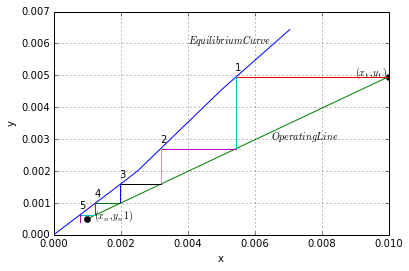## Example 12.8-1 Page Number 726¶

In :
#Prediction of Time for Batch Leaching

#Variable Declaration
Es = 0.2             #Fraction unextracted
d1 = 2.0             #Initial particle diameter, mm
d2 = 1.5             #Initial particle diameter, mm
t1 = 3.11            #Time in hr
#Calculation
# Using figure 5.3-13 page 349 for sphere Es = 0.2 alpha*t/a**2 = 0.112 it is same in both sizes
absc = 0.112
a1 = d1/2
a2 = d2/2
t2 = t1*a2**2/a1**2

#Results
print "Time require for 80% separation wich changed size",round(t2,2),'h'

Time require for 80% separation wich changed size 1.75 h


## Example 12.9-1 Page Number 731¶

In :
#Single Stage Leaching of Flaked Soyabeans
import numpy as np
import matplotlib.pylab as plt
#Variable Declaration
V2 = 100.0        #Fresh Solvent, kg
F = 100.0         #Feed of Soyabeen flakes, kg
xA2 = 0.0         #Compositions in wt fractions
xC2 = 1.0
y0 = 0.20
yA0 = 1.0
Nn = 1.5          #kg insoluble rtained/ jgsolution retained

#Calculation
B = F*(1.0-y0)
L0 = F*y0
N0 = B/L0
M = L0 + V2
# Mxam = L0*y0 + V2*xA2
xAm = L0/M
#B = Nm*M
Nm = B/M
xA = np.array([0.0,0.2,0.4,0.6,0.8,1.0])
yA = np.array([0.0,0.2,0.4,0.6,0.8,1.0])
N = np.array([0,1,2,3,4,5])
plt.grid(True)
plt.plot([0,yA0],[0.0,N0])
plt.xlabel("$x_A,y_A$")
plt.ylabel("$N$")
plt.ylabel("$N$")
plt.plot([0.,1.0],[Nn,Nn])
plt.plot([xAm,xAm],[0.0,Nn])
plt.text(0.6,0.1," $N$ vs $x_A$ ")
plt.text(0.6,1.6," $N$ vs $y_A$ ")
plt.text(yA0,N0," $L_0$ ")
plt.plot(yA0,N0,'ro')
plt.text(xAm,Nm," $M$ ")
plt.plot(xAm,Nm,'ro')
N1 = 1.5
yA1 = xAm
xA1 = xAm
plt.text(xA1,0," $V_1$ ")
plt.plot(xA1,N1,'ro')
m = (N-N)/(xA-xA)
yAm = m*xAm
plt.text(xA1,N1," $L_1$ ")
plt.plot(xA1,Nm,'ro')
plt.plot(xA2,0,'ro')
l = (Nn-0.0)
l1 = (yAm-0.0)
l2 = (Nn-yAm)
V1 = M*l1/l
L1 = M*l2/l

#Results
print 'Equilibrium amounts of L1, V1 are %3.1f and %3.1f kg'%(L1,V1)

Equilibrium amounts of L1, V1 are 53.3 and 66.7 kg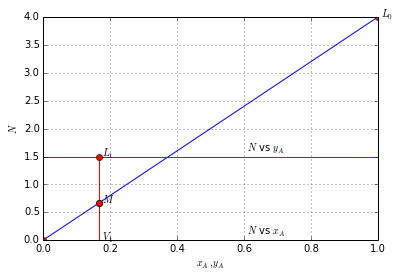## Example 12.10-1 Page Number 735¶

In :
#Counterurrent Extraction of Oil from meal
import numpy as np
from scipy.optimize import curve_fit, root
import matplotlib.pyplot as plt
from scipy.interpolate import interp1d

#Variable Declaration
X = []
N = np.array([2.00,1.98,1.94,1.89,1.82,1.75,1.68,1.61])   #kg of inert solid B/kg solution
yA = np.array([0.0,0.1,0.2,0.3,0.4,0.5,0.6,0.7])          #kg of inert oil A/kg solution
FMi = 2000.      #Rate of Mass of inert solid meal, kg/hr
FMo = 800.       #Rate of Mass of oil, kg/hr
FMb = 50.        #Rate of Mass of Benzene with feed, kg/hr
FSb = 1310.      #Rate of Mass of Benzene with fresh solvent, kg/hr
FSo = 20.        #Rate of Mass of oil with fresh solvent, kg/hr
LS = 120.        #Rate of Mass of Leached solids, kg/hr

#Calculation
L0 = FMo+FMb
yA0 = FMo/L0
B = FMi
N0 = B/L0
Vn1 = FSb + FSo
xAn1 = FSo/Vn1
M = L0 + Vn1
xAM = (L0*yA0 + Vn1*xAn1)/M
NM = B/M
sL0Vn1 = B/LS

cL0Vn1 = NM - sL0Vn1*xAM
xx = -cL0Vn1/sL0Vn1

plt.grid(True)
plt.xlabel('$x_A$  $y_A$')
plt.ylabel('$N$')
plt.plot(yA0,N0,'ro')           #plot L0
plt.text(yA0,N0,'$L_0$')
plt.plot(0.0,0.0,'ro')          #plot origin
plt.plot(xAn1,0.0,'bo')         #Plot Vn+1
plt.text(-xAn1-0.06,0.2,'$V_{N+1}$')
plt.plot([xAn1,yA0],[0.0,N0])   #plot line L0 to Vn+1
plt.plot(xAM,NM,'ro')           #plot M
plt.text(xAM-0.05,NM-0.5,'$M$')
plt.plot(yA,N,'b-')             #Plot N vs yAN+1
plt.text(yA[len(yA)-1],N[len(yA)-1],'$N$ $vs$ $y_A$')

ff= lambda x:f(x)-sL0Vn1*x
sol = root(ff,0.01)
yAn = sol.x
Nn = f(yAn)

plt.plot([0.0,yAn],[0.0,Nn])
plt.plot([xAn1,yAn],[0.0,Nn])    #Plot Vn+1 to Ln

s1 = (NM-Nn)/(xAM-yAn)
c1 = NM-s1*xAM
x1 = -c1/s1
X.append(x1)
plt.plot([x1,yAn],[0.0,Nn])      #Plot Ln to V1
sdL0 = (N0-0.0)/(yA0-x1)
c2 = -sdL0*x1

s3 = (Nn-0.0)/(yAn-xAn1)
c3 = -s3*xAn1
delx = (c3-c2)/(sdL0-s3)
dely = sdL0*delx+c2
plt.text(delx,dely-0.5,'$\delta$')
plt.plot([yA0,delx],[N0,dely])     #Draw a line from V1 to delta
plt.plot([xAn1,delx],[0.0,dely])   #Draw a line from Vn+1 to delta
plt.plot([1.0,-0.4],[0.0,0.0])
x = x1
j = 0
while x > xAn1:
j = j+ 1
y = f(x)
plt.plot(x,0.0,'bo')
plt.text(x,-0.5,'V'+str(j))
plt.plot([x,x],[0.0,y])            #Move to N vs y curve
plt.plot(x,y,'bo')
plt.text(x,y+0.5,'L'+str(j))
plt.plot([x,delx],[y,dely])        #from x,y Draw a line joining to delx,dely
slope = (y-dely)/(x-delx)
inter = y - slope*x
xnew = -inter/slope
X.append(xnew)
x = xnew

a = np.array([[1,1], [yAn,X] ])
b = np.array([M,M*xAM])
Ln,V1 = np.linalg.solve(a, b)

#Results
print 'Rate of leached solution %5.1f kg/h'%Ln
print 'Composition of leached solution %5.4f kg/h'%yAn

print 'Rate of solvent out %5.1f kg/h'%V1
print 'Composition of solvent out %5.3f kg/h'%X

print 'Rate of leached solids %8.1f kg solution/h' %(M)
print "Number of equilibrium stages required are",j

Rate of leached solution 1014.0 kg/h
Composition of leached solution 0.1183 kg/h
Rate of solvent out 1166.0 kg/h
Composition of solvent out 0.600 kg/h
Rate of leached solids   2180.0 kg solution/h
Number of equilibrium stages required are 4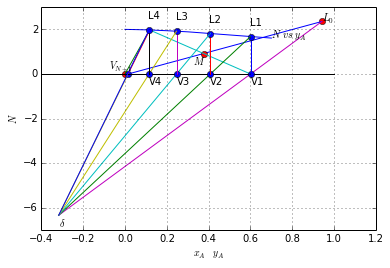## Example 12.11-1 Page Number 739¶

In :
#Yield of Crystalization Process
import numpy as np
from numpy.linalg import solve

#Variable Declaration
F = 10000.           #Weight of salt solution, kg
xFc = 0.30           #Weight percent of salt
Ts = 293.            #Temperature of salt solution, K
S = 21.5             #Unhydrous Solubility, kg/100 kg of water
MW = 106.0           #Molecular wt of Na2CO3
MWh = 180.2          #Molecular wt of 10.H2O

#Calculation
MWhs = MW + MWh      #Molecular wt of Na2CO3.10.H2O
xFs = 1.0 - xFc
xWs,xWc = 1.0, 0.0

xCs,xCc = MWh/MWhs,MW/MWhs
xSs,xSc = 100/(S+100),S/(S+100)

#Part A
W = 0.0
a = np.array([[1,1], [xSs,xCs]])
b = np.array([F-W, F*xFs-W*xWs])
[Sa,Ca] = solve(a,b)

#Part B
W = F*0.03
a = np.array([[1,1], [xSc,xCc]])
b = np.array([F-W, F*xFc-W*xWc])
[Sb,Cb]= solve(a, b)

#Results
print "kgs of Hydrayred Crystal produced and Saturated solution are", round(Ca,1),"&", round(Sa,1),"respectively"
print "kgs of Hydrayred Crystal produced and Saturated solution are", round(Cb,1),"", round(Sb,1),"respectively"
print 'The results are checked by using tools like calculator and\nother the results of this code are more correct than book'

Answer to PART A
kgs of Hydrayred Crystal produced and Saturated solution are 6361.7 & 3638.3 respectively
kgs of Hydrayred Crystal produced and Saturated solution are 6636.2  3063.8 respectively
The results are checked by using tools like calculator and
other the results of this code are more correct than book


## Example 12.11-2 Page Number 741¶

In :
#Heat Balance in Crystallization

from numpy.linalg import solve

#Variable Declaration
F = 2268.0             #kg of feed solution
TF = 327.6             #Temperature of feed solution, K
CF = 48.2              #kgMgSO4/100 kg water
Tc = 293.2             #Temperature cooled to, K
S = 35.5               #kgMgSO4/100 kg water
cp = 2.93              #Average heat Capcity of Solution at 298.2 K, kJ/kg.K
delHs = -13.31e3       #Heat of solution at 298.2 K, kJ/kmol
MW = 120.368           #MW of MgSO4
MWwh = 126.107         #MW of Water of Hydration 7H2O

#Calculation
xFs = 100./(100+CF)
xFc = 1.0 - xFs
xSs = 100./(100. + S)
xSc = 1.0 - xSs
xCs = MWwh/(MW + MWwh)
xCc = 1.0 - xCs

a = numpy.array([[1.,1.], [xSc,xCc]])
b = numpy.array([F, F*xFc])
[So,C]= solve(a, b)

H1 = F*(TF-Tc)*cp
delHs = delHs/(MW+MWwh)
delHc = -(delHs)
Hc = delHc*C
Q = - Hc - H1
#Results
print 'Mass of Hydrayred Crystal produced and Saturated solution are %5.2f & %5.2f kg'%(C,So)
print "Total heat Absorbed", round(Q,2), "kJ. Negative sign indicates heat must be removed"
print 'The results are checked by using tools like calculator and\nother the results of this code are more correct than book'

Mass of Hydrayred Crystal produced and Saturated solution are 633.65 & 1634.35 kg
Total heat Absorbed -262814.27 kJ. Negative sign indicates heat must be removed
The results are checked by using tools like calculator and
other the results of this code are more correct than book